# Design and Analysis of the STATCOM Based on Diode Clamped Multilevel Converter Using Model Predictive Current Control Strategy

Design and Analysis of the STATCOM Based on Diode Clamped Multilevel Converter Using Model Predictive Current Control Strategy
Middle Technical University, Electrical Engineering Technical college, Baghdad 10001, Iraq

Corresponding Author Email:
sabahsuha9@gmail.com
Page:
221-228
|
DOI:
https://doi.org/10.18280/ejee.230306
4 June 2020
|
Accepted:
16 April 2021
|
Published:
21 June 2021
| Citation

OPEN ACCESS

Abstract:

In recent decades, multi-level converters have become popular and used in many power systems applications. Compared with conventional converters, multi-level converters contribute to reducing the voltage stress on the switching devices and enhancing the power quality delivered to the load. In this paper, the study of the five-level diode clamped multilevel converter based static synchronous compensator has been accomplished. Model Predictive current control strategy which a type of modern control algorithms was employed for driving the proposed compensator. The suggested five level converter controlled by model predictive current control is firstly examined to verify that this control algorithm is appropriate for achieving the desired performance. Then the proposed converter and control combination is employed and simulated as a static synchronous compensator in distributed power system. Moreover, in order to examine the robustness of this compensator, the load status is suggested to be heavy inductive. Simulation process has been performed using MATLAB – SIMULINK software package. The results show that the implemented configuration (converter and control algorithm) provides high power quality improvement with adequate reactive power compensation.

Keywords:

STATCOM, diode clamped converter, model predictive current control, low harmonic distortion

1. Introduction

In this paper MPCC will be employed for driving the 3-phase five-level DCMLC firstly as an inverter afterward it will be used for driving the STATCOM based on five-level DCMLC. In this paper, the basic principles of the Diode Clamped Multi-level converter are covered in section 2. The Model Predictive Current Control strategy and the derivation of the discrete-time model of the converter were explained in section 3. The principles of the STATCOM are briefly demonstrated in section 4, while its configuration in power system is explained in section 5. In section 6, the simulation results for the inverter and the STATCOM are presented. Finally, the conclusions are outlined in section 7.

2. Diode Clamped Multi-Level Converter DCMLC

This type of converters has been firstly introduced in 1979  and then it is popularized in 1980’s [17, 18], and it is recently considered as the basic structure of the recent multilevel converters . It has been employed for high voltage and high-power applications for many years . For n-level DCMLC, the DC voltage source (Vdc) is uniformly partitioned into a smaller level through (n -1) series connected capacitors which leads to stepped the output voltage of the converter and limits the voltage stress on the switching device to the Vdc/(n-1) . Also, DCMLC requires (n-1) × (n-2) clamping diodes/ phase with equally voltage ratings [17–19]. As the number of the voltage levels are increased, high quality power delivered to the load is obtained and the load voltage shape becomes closer to the sine waveform . In this paper, 5- level DCMLC (FLDCMLC) is proposed, one leg FLDCMC is shown in Figure 1, the circuit contains of 8-switching devices, 12-clamping diodes and 4-capacitors, C1, C2, C3 and C4. Suppose that the magnitude of the dc supply voltage is Vdc, then the voltage through each capacitor is Vdc/4. Therefore, each power switch voltage stress will be restricted to the level of Vdc/4 by means of clamping diodes. Table 1 shows the switching states and the corresponding voltages for one leg of the converter.

## 1.png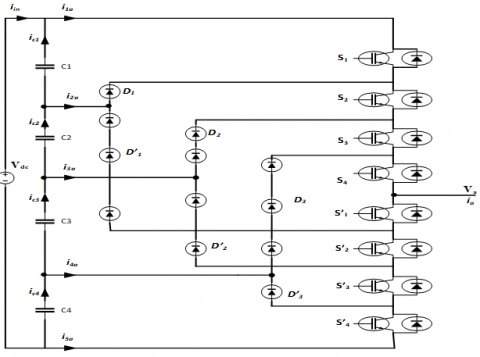Figure 1. Structure of Five Level Diode Clamped Multi-Level Converter FLDCMLC

Table 1. Switching states and corresponding voltages of FLDCMC per leg

 Converter States/leg x Voltage (Vx) S1 S2 S3 S4 S1՛ S2՛ S3՛ S4՛ 0 0 1 1 1 1 0 0 0 0 1 1 1 1 0 0 0 +Vdc /4 1 1 1 1 0 0 0 0 +Vdc /2 0 0 0 1 1 1 1 0 -Vdc /4 0 0 0 0 1 1 1 1 -Vdc /2

The total switching states of FLDCMC are 125 states, while there are some states are redundant and produce the same voltage vector, therefore the converter can apply 61 different voltage vectors to the load from the total number of switching states . For three-phase applications, the voltage vector produced based on each switching state is determined according to the following equation:

$v=\frac{2}{3}\left(v_{a}+\boldsymbol{a} v_{b}+\boldsymbol{a}^{2} v_{c}\right)$    (1)

where, a is the complex operator: $\boldsymbol{a}=e^{j 2 \pi / 3}$, va, vb and vc are the voltages obtained according to Table 1.

3. Model Predictive Current Control Strategy

Model predictive current control (MPCC) is a very vast type of control algorithms that is found comparatively recent in the power converters applications. The major feature of MPCC is the investment of the system model to predict the future action of the controlled variables. The control algorithm makes use of this information to produce the optimal actuation pulses based on the predefined index [11, 14, 16, 22]. The MPCC based control of the FLDCMLC which is connected to the 3-phase RL load through RL filter is shown in Figure 2. The prediction process is performed for all switching states combinations per sampling interval and the switching state that produce minimum cost function will be delivered to the output at the adjacent sampling period .

## 2.png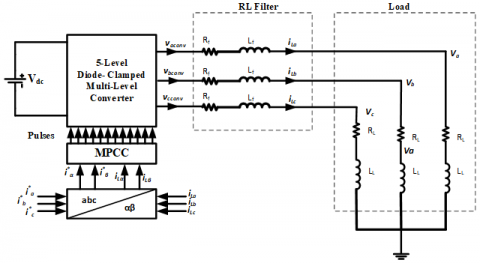Figure 2. MPCC based FLDCMLC control

Three phase reference and measured line currents are transformed to the alpha – beta axes using Clarke transformation method which can be described as shown in Eq. (2) below:

$\left[\begin{array}{l}i_{\alpha}(t) \\ i_{\beta}(t)\end{array}\right]=\frac{2}{3}\left[\begin{array}{ccc}1 & -\frac{1}{2} & -\frac{1}{2} \\ 0 & \frac{\sqrt{3}}{2} & -\frac{\sqrt{3}}{2}\end{array}\right]\left[\begin{array}{l}i_{a}(t) \\ i_{b}(t) \\ i_{c}(t)\end{array}\right]$    (2)

The differential equation at the load side of the circuit is obtained using Kirchhoff's voltage law (KVL) as in Eq. (3):

$v(t)-L_{L} \frac{d i(t)}{d t}-R_{L} i(t)-e(t)=0$    (3)

where, v(t) is the complex form of the converter voltage obtained from Eq. (1) , e(t) is the back-emf induced in the load circuit and i(t) is the complex form of the load current which has the following form:

$i(t)=i_{\alpha}(t)+j i_{\beta}(t)$    (4)

Eq. (3) can be re-arranged as follows:

$\frac{d i(t)}{d t}=\frac{1}{L_{L}}(v(t)-e(t))-\frac{R_{L}}{L_{L}} i(t)$    (5)

The discrete-time form of the load current can be described using 1st order forward Euler’s equation:

$\frac{d i(t)}{d t}=\frac{i(k+1)-i(k)}{T_{s}}$    (6)

where, i(k) is the load current at sample k, i(k+1) is the load current at sample (k+1) and $T_{s}$ is the sampling interval. Therefore, the resulting discrete-time form of Eq. (4) will be:

$i^{p}(k+1)=\left(1-\frac{R_{L} T_{s}}{L_{L}}\right) i(k)+\frac{T_{s}}{L_{L}}\left(v(k)-e^{\wedge}(k)\right)$    (7)

It is clear from Eq. (6) that the future value of the load current can be predicted according to the parameters obtained at the current sample. The estimated value of the back-emf ($e^{\wedge}(k)$) can be determined from the following equation:

$e^{\wedge}(k-1)=v(k-1)-\left(\frac{L_{L}}{T_{s}}\right) i(k)$

$-\left(R_{L}-\frac{L_{L}}{T_{s}}\right)(i(k-1)$    (8)

The determination of the cost function is described by the following equation:

\begin{aligned} g=\mid i_{\alpha}^{*}(k+1)-i & i_{\alpha}^{p}(k+1) \mid \\ &+\left|i_{\beta}^{*}(k+1)-i_{\beta}^{p}(k+1)\right| \end{aligned}    (9)

where, $i_{\alpha}^{*}$ is the real component of reference current, $i_{\beta}^{*}$ is the imaginary component of the reference current, $i_{\alpha}^{p}$ is the real component of predicted current and $i_{\beta}^{p}$ is the imaginary component of predicted current at the adjacent sampling interval (k+1). Reference current is obtained from outer loop and the load current at sample interval (k) is measured, then the model will estimate the value of load current at (k+1) for each switching state (voltage vector), and the cost function is determined.

The flow chart shown in Figure 3 summarize the MPCC algorithm for controlling the FLDCMC :

## 3.png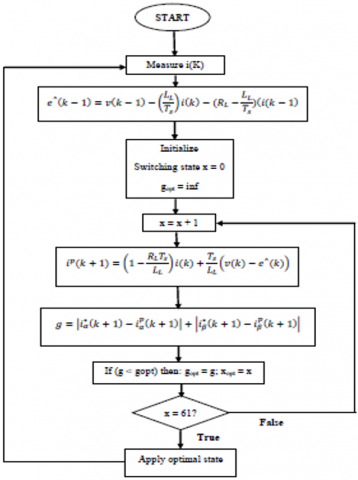Figure 3. Flow chart of MPCC for FLDCMLC

The initial conditions are the state of the converter and the cost function. The state of the converter which produces zero output voltage was taken as the starting point for each sampling interval. Moreover, for each sampling interval the cost function is assumed to be infinity to guarantees the convergence to the minimum value.

4. STATCOM Principles

STATCOM is a power electronics voltage source converter employed to inject or absorb reactive power into the electrical grid. It is a member of Flexible AC Transmission System (FACTS) family shunt devices . Figure 4 shows the basic connection of the STATCOM to the electric power grid through step-down transformer which is replaced by its equivalent circuit and as an RL filter. The STATCOM block contains the FLDCMLC and MPCC that explained previously in Figure 2, the DC source is eliminated in this case. The principles of the reactive power exchange between STATCOM and the grid is illustrated in Figure 5. At steady state Vbus = Vconv and no reactive power (Q) exchange will occur, when the Vbus > Vconv the reactive power (Q) will flow from Bus to the STATCOM (absorption) and when the Vbus < Vconv then the reactive power (Q) will flow from STATCOM to the Bus (injection). The exchanging process can be described by the following equation :

$Q=\frac{V_{\text {conv }}\left(V_{\text {conv }}-V_{\text {bus }}\right)}{X_{f}}$    (10)

The differential equation at the AC side of the circuit of Figure 4 is obtained using Kirchhoff's voltage law (KVL):

$v_{s}(t)-L_{f} \frac{d i_{c}(t)}{d t}-R_{f} i_{c}(t)-v_{c}(t)=0$    (11)

where, $v_{s}(t)$ is the bus voltage in complex form ($v_{s \alpha}(t)$ and $v_{s \beta}(t)$) and $v_{c}(t)$ is the STATCOM voltage in complex form according to Eq. (1). The discrete-time model of Eq. (10) based on 1st order Euler’s equation will be:

$i_{c}^{p}(k+1)=\frac{T_{s}}{L_{f}}\left(v_{s}(k)-v_{c}(k)\right)+\left(1-\frac{R_{f} T_{s}}{L_{f}}\right) i_{c}(k)$    (12)

When it is working with STATCOM, MPCC algorithm will adopt Eq. (12) instead of Eq. (7) and Eq. (8) will be not used.

5. STATCOM Configuration in Power System

The above – mentioned STATCOM based on FLDCMLC is configured for compensating the power quality delivered to the loads of the distribution power system shown in Figure 6. This power system is connected in a ring manner and its parameters are listed in Table 2 shown below:

Table 2. Distribution power system parameters

 Description of Parameters Rating Rated voltage 11 KV Base voltage 11 KV Frequency 50 Hz S.C level 500 MVAR Load 1 300 MW, 30 MVAR Load 2 300 MW, 30 MVAR Load 3 200 MW, 20 MVAR Load 4 200 MW, 20 MVAR

## 4.pngFigure 4. Basic connection of STATCOM

## 5.png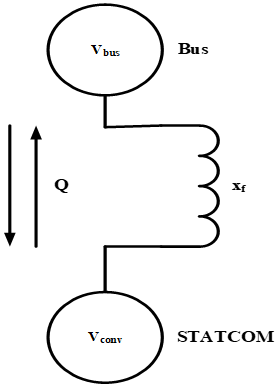Figure 5. Principles of STATCOM operation

## 6.pngFigure 6. STATCOM based on FLDCMC

6. Simulation Results

The performance of the proposed five-level inverter (FLDCMLC) and the STATCOM based on FLDCMLDC have been examined by simulation using MATLAB / SIMULINK software package. The simulation of the five-level inverter is carried out based on Figure 2 and the parameters listed in Table 3.

Simulation results of the FLDCMLC is shown in Figure 7. It is clear from these results that the requirements for high quality and low distortion of the power system for load demands have been achieved. Figure 7(a) shows a three-phase line-to-line output voltage of the inverter with Total Harmonic Distortion (THD) of 18.1%. A very low THD (0.48%) is obtained of the filtered three-phase line-to-line output voltage as shown in Figure 7(b). Figure 7(c) shows the line-to-neutral load voltage and load current with THD of 0.59% for both. Also, it can be noticed that the power factor is very close to unity.

Table 3. FLDCMC Parameters

 Description of Parameters Rating NO. of switches / arm 8 Dc Capacitor 10 mF DC-Link voltage 500 V frequency 50 Hz Phase voltage peak value 480 V Filter inductor 22 mH Filter capacitor 500 μF RL load / Phase 18 Ω and 1 mH

## 7a.png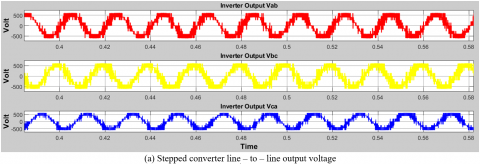## 7b.png## 7c.png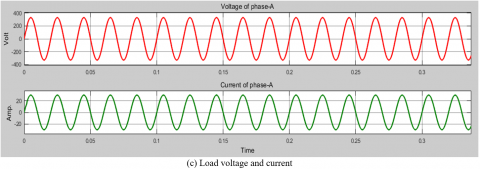Figure 7. FLDCMLC waveforms

## 8a.png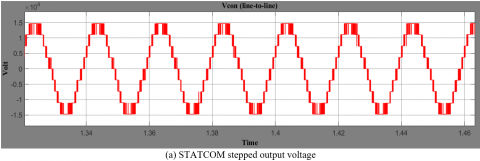## 8b.png## 8c.png## 8d.pngFigure 8. STATCOM performance

For STATCOM based on FLDCMLC the simulation process is performed based on Table 1 and Figure 6, the results obtained are shown in Figure 8, a stepped output voltage has been acquired from the STATCOM as indicated in Figure 8(a) with acceptable THD (15.2%). The STATCOM supplied a filtered output voltage to the grid with a significant reduction in THD (0.3%) as shown in Figure 8(b). The compensation performance of the suggested STATCOM is verified as shown in Figure 8(c). The top part of Figure 8(c) shows the output voltage response of the STATCOM in p.u. The second part of this figure displays the compensation process of the quadrature component (Iq) of the output current compared with reference one. The reactive power compensation process was appeared in the third part of Figure 8(c), and the angle difference (delta) between the grid voltage and STATCOM voltage is shown bottom part of this figure. A balance voltage across the capacitors of the STATCOM are shown in Figure 8(d), it can be noticed that the DC voltage is divided evenly across the capacitors.

7. Conclusion

This paper study the operation of the five-level Diode Clamped multi-level converter as an inverter for driving inductive load and as a STATCOM for compensating the voltage at the electrical distribution system. Model Predictive Current Control strategy was adopted for controlling the STATCOM. Simulation results show that the five-level Diode Clamped multi-level inverter supplies a high-quality ac power by means of a significant reduction in the Total Harmonic Distortion (THD) with a close to unity power factor. The stepped form of the output voltage of the inverter indicates the possibility of constraining the voltages across the devices. Therefore, multi-level converter configurations permit of using switching devices in the higher voltages’ ratings. These encouraging results have made the use of multi-level converters to work as STATCOM. The STATCOM based on the FLDCMLC was adopted for compensating the power quality of the electrical power distribution system. The performance of the proposed STATCOM was examined by simulation and the results show the fast response of the STATCOM to compensate the power quality of the grid. Adoption of the model predictive current control for controlling the converter and the STATCOM significantly simplified the control structure and calculations. This control strategy provides a fast response with maintaining a high quality of the load voltages and currents.

Nomenclature
 a Complex operator $e^{j 2 \pi / 3}$ $e^{\wedge}$ g Estimated back emf Cost function i $i^{*}$ Measured current, Amp Reference current, Amp $i^{p}$ k v va,b,c Vdc x Predicted current, Amp Current sample Voltage vector, volt Three-phase voltages, volt DC voltage applied to the inverter, volt Switching state Greek symbols $\alpha$ Stationary axis aligned with phase A $\beta$ Stationary axis orthognal with $\alpha$
References

 Pirouz, H.M., Bina, M.T. (2009). New transformerless STATCOM topology for compensating unbalanced medium-voltage loads. In 2009 13th European Conference on Power Electronics and Applications, pp. 1-9.

 Adepoju, G.A., Komolafe, O.A. (2011). Analysis and modelling of static synchronous compensator (STATCOM): A comparison of power injection and current injection models in power flow study. International Journal of Advanced Science and Technology, 36(2): 65-76.

 Nwobu, C.J., Efika, I.B., Oghorada, O.K., Zhang, L. (2015). A modular multilevel flying capacitor converter-based STATCOM for reactive power control in distribution systems. In 2015 17th European Conference on Power Electronics and Applications (EPE'15 ECCE-Europe), pp. 1-9. https://doi.org/10.1109/EPE.2015.7309085

 Soto, D., Green, T.C. (2002). A comparison of high-power converter topologies for the implementation of FACTS controllers. IEEE Transactions on Industrial Electronics, 49(5): 1072-1080. https://doi.org/10.1109/TIE.2002.803217

 Mohammadi, P.H., Bina, M.T. (2010). A transformerless medium-voltage STATCOM topology based on extended modular multilevel converters. IEEE Transactions on Power Electronics, 26(5): 1534-1545. https://doi.org/10.1109/TPEL.2010.2085088

 Liu, X., Lv, J., Gao, C., Chen, Z., Chen, S. (2016). A novel STATCOM based on diode-clamped modular multilevel converters. IEEE Transactions on Power Electronics, 32(8): 5964-5977. https://doi.org/10.1109/TPEL.2016.2616495

 Leon, J.I., Vazquez, S., Franquelo, L.G. (2017). Multilevel converters: Control and modulation techniques for their operation and industrial applications. Proceedings of the IEEE, 105(11): 2066-2081. https://doi.org/10.1109/JPROC.2017.2726583

 Rodriguez, J., Kazmierkowski, M.P., Espinoza, J.R., Zanchetta, P., Abu-Rub, H., Young, H.A., Rojas, C.A. (2012). State of the art of finite control set model predictive control in power electronics. IEEE Transactions on Industrial Informatics, 9(2): 1003-1016. https://doi.org/10.1109/TII.2012.2221469

 Antonopoulos, A., Angquist, L., Nee, H.P. (2009). On dynamics and voltage control of the modular multilevel converter. In 2009 13th European Conference on Power Electronics and Applications, pp. 1-10.

 Hagiwara, M., Akagi, H. (2009). Control and experiment of pulse width-modulated modular multilevel converters. IEEE Transactions on Power Electronics, 24(7): 1737-1746. https://doi.org/10.1109/TPEL.2009.2014236

 Geyer, T., Darivianakis, G., Van Der Merwe, W. (2015). Model predictive control of a STATCOM based on a modular multilevel converter in delta configuration. In 2015 17th European Conference on Power Electronics and Applications (EPE'15 ECCE-Europe), pp. 1-10. https://doi.org/10.1109/EPE.2015.7309148

 Aurtenechea, S., Rodriguez, M.A., Oyarbide, E., Torrealday, J.R. (2007). Predictive direct power control of MV-grid-connected two-level and, three-level NPC converters: experimental results. In 2007 European Conference on Power Electronics and Applications, pp. 1-10. https://doi.org/10.1109/EPE.2007.4417305

 Barros, J.D., Silva, J.F. (2009). Multilevel optimal predictive dynamic voltage restorer. IEEE Transactions on Industrial Electronics, 57(8): 2747-2760. https://doi.org/10.1109/TIE.2009.2034172

 Cortés, P., Kazmierkowski, M.P., Kennel, R.M., Quevedo, D.E., Rodríguez, J. (2008). Predictive control in power electronics and drives. IEEE Transactions on Industrial Electronics, 55(12): 4312-4324. https://doi.org/10.1109/TIE.2008.2007480

 Rodriguez, J., Pontt, J., Cortés, P., Vargas, R. (2005). Predictive control of a three-phase neutral point clamped inverter. In 2005 IEEE 36th Power Electronics Specialists Conference, pp. 1364-1369. https://doi.org/10.1109/PESC.2005.1581807

 Vazquez, S., Leon, J.I., Franquelo, L.G., Rodriguez, J., Young, H.A., Marquez, A., Zanchetta, P. (2014). Model predictive control: A review of its applications in power electronics. IEEE Industrial Electronics Magazine, 8(1): 16-31. https://doi.org/10.1109/MIE.2013.2290138

 Cho, G.H. (1991). A general circuit topology of multilevel inverter. PESC '91 Record 22nd Annual IEEE Power Electronics Specialists Conference, Cambridge, MA, USA.

 Kim, Y.S., Seo, B.S., Hyun, D.S. (1993). A novel structure of multi-level high voltage source inverter. In 1993 Fifth European Conference on Power Electronics and Applications, pp. 132-137. https://doi.org/10.1109/TENCON.1993.320692

 Raj, P.H., Maswood, A.I., Ooi, G.H., Lim, Z. (2015). Voltage balancing technique in a space vector modulated 5-level multiple-pole multilevel diode clamped inverter. IET Power Electronics, 8(7): 1263-1272. https://doi.org/10.1049/iet-pel.2014.0747

 Khajehoddin, S.A., Bakhshai, A., Jain, P.K. (2006). Modeling and analysis of 5-level diode-clamped and flying-capacitors converters. In 2006 IEEE International Symposium on Industrial Electronics, 2: 1408-1413. https://doi.org/10.1109/ISIE.2006.295678

 Dhal, P.K., Rajan, C.C.A. (2015). Design and comparative analysis of diode clamped multilevel inverter for eliminating total harmonics using high switching frequency techniques. In Power Electronics and Renewable Energy Systems, 665-677. https://doi.org/10.1007/978-81-322-2119-7_65

 Kieferndorf, F., Karamanakos, P., Bader, P., Oikonomou, N., Geyer, T. (2012). Model predictive control of the internal voltages of a five-level active neutral point clamped converter. In 2012 IEEE Energy Conversion Congress and Exposition (ECCE), pp. 1676-1683. https://doi.org/10.1109/ECCE.2012.6342611

 Hassan, R.F. (2018). Design and software implementation of solid state transformer. International Journal of Engineering & Technology, 7(3): 1776-1782. https://doi.org/10.14419/ijet.v7i3.16423

 Rivera, M., Rodriguez, J., Vazquez, S. (2016). Predictive control in power converters and electrical drives-Part i. IEEE Transactions on Industrial Electronics, 63(6): 3834-3836. https://doi.org/10.1109/TIE.2016.2549498

 Ali, H., Rahardjo, A., Setiawan, A., Jufri, F.H., Husnayain, F. (2019). Voltage profile improvement analysis during the loss of transmission lines on 150kv subsystem using static synchronous compensator. In IOP Conference Series: Materials Science and Engineering, 673(1): 012066. https://doi.org/10.1088/1757-899X/673/1/012066